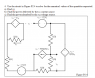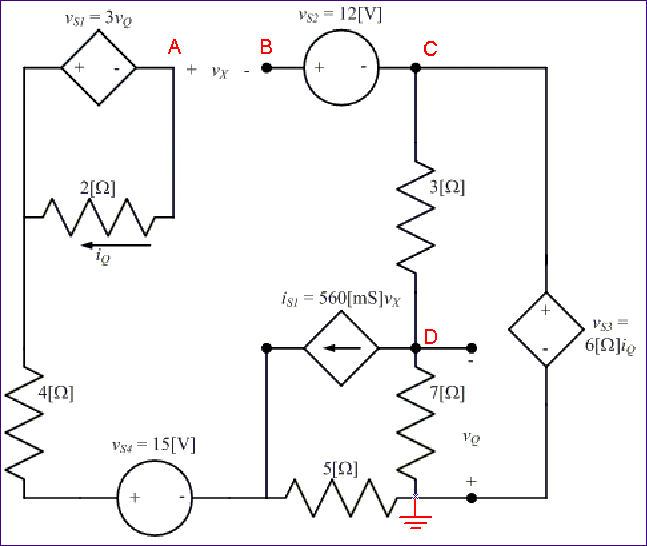# Solving for multiple unkowns using KVL and KCL equations

• fredro

## Homework Statement

I am asked to solve for vX, PDEV BY iS1, P ABS BY vS3 (please see attached figure). We have only covered KCL, KVL, Ohm's law and Power in class. No Thevinen theorem, so it can't be used to help solve.

## The Attempt at a Solution

I know that current will not pass through the 4 Ohm resistor or vS4. My initial hunch was to solve for iQ in terms of vS1 (upper left of figure) and then use that equation to help solve for a KVL with the 3 and 7 ohm resistors and vS3. The result was not pretty and very wrong.

#### Attachments

•Circuit 1.png
10.8 KB · Views: 582

Yeah, it's going to get messy. There are a lot of interrelationships to account for with all the controlled sources. However, to make a start, choose a ground node, label the other important nodes, and see what you can do about writing equations for their voltages in terms of the given information. Here's a suggestion:The voltage at node D (which is the same as -vQ) will be important. You can write a KCL equation for that node.

#### Attachments

Here's what I have so far. Unfortunately, I keep running into nonsensical, trivial answers where my systems keep having coefficients of zero.I've spent several hours on this problem.

#### Attachments

Watch out for the polarities of things. The diagram shows vQ to be the negative of the voltage at your KCL1 node (note the +/- sign locations).

Suppose you take the voltage at node D to be vD. Then $v_Q = -v_D$. Can you write expressions for the node voltages at A and B (with respect to the ground node that I indicated) in terms of this vD ? (You may need to keep vx as a variable for now).

Here's what I have so far. Unfortunately, I keep running into nonsensical, trivial answers where my systems keep having coefficients of zero.I've spent several hours on this problem.